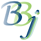# BBjVector::size

## Description

Returns the number of items in vector.

Return Value

Method

int

size()

None.

## Return Value

Returns the number of items in the vector.

None.

## Example

 ``````rem 'Get the size of a BBjVector object rem 'Obtain the instance of the BBjAPI object let myAPI! = BBjAPI() rem 'Create an instance of the BBjVector object let myVector! = myAPI!.makeVector() rem 'Create values to add into the BBjVector A! = new java.lang.Integer(1) A = 2 B! = new java.lang.String("Item 3") B\$ = "Item 4" rem 'Add the above values into the BBjVector object myVector!.addItem(A!) myVector!.addItem(A) myVector!.addItem(B!) myVector!.addItem(B\$) rem 'Get the number of items in the vector, this returns 4 VECTOR_SIZE = NUM(myVector!.size())``````